# How to Integrate Compiled MATLAB Functions with LabVIEW with a .NET Assembly Approach

Publish Date: Jul 28, 2020 | 0 Ratings | 0.00 out of 5 | Print | Submit your review

## Overview

This tutorial utilizes The MathWorks, Inc. MATLAB®, MATLAB Compiler™, and MATLAB Compiler SDK™ to create a .NET assembly that can be called in LabVIEW. This approach greatly reduces overhead and improves runtime compared to other methods such as calling executables or MATLAB script node.

Integrating MATLAB to LabVIEW enables complete reuse of MATLAB code. At the end of the tutorial, scientists and engineers who use both platforms should be able to seamlessly integrate MATLAB in LabVIEW.

### 1. Software Requirements

1. LabVIEW Development System
2. MATLAB,  MATLAB Compiler™, and MATLAB Compiler SDK™
3. On the deployment machines you need to install MATLAB Runtime

Note: The version of MATLAB Runtime should be the same as the version of MATLAB that was used to create the compiled MATLAB code.

### 2. Generate a .NET Assembly from MATLAB with Library Compiler App

1. Generate a .NET assembly from MATLAB using the Library Compiler App. The tutorial from MathWorks, Inc. uses the makesquare.m example file and results in the creating of MagicSquareCompNative.dll, which is the file we will be using to integrate into LabVIEW in this tutorial.
2. Verify the generated folders and files. Three folders are generated in the target folder location: for_redistribution, for_redistribution_files_only, and for_testing.
We will be using MagicSquareCompNative.dll in for_testing folder for the next step.
Note:
We use MagicSquareCompNative.dll rather than MagicSqaureComp.dll because  MagicSqaureComp.dll uses M array data type and this data type is not supported in LabVIEW.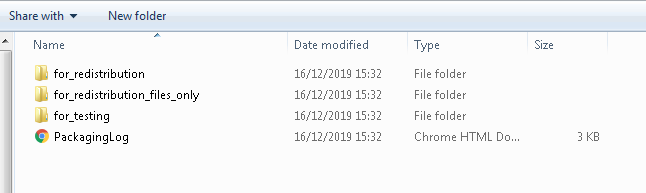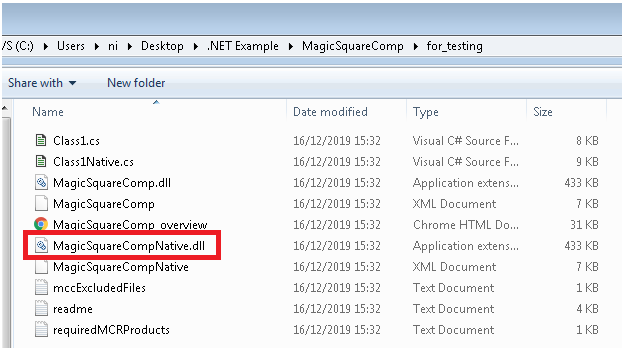### 3. Call .NET Assembly from LabVIEW

1. Open a new VI by launching LabVIEW >> File >> New VI.
2. On the block diagram, add a Constructor Node from the Connectivity >> .NET pallet.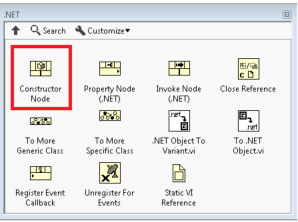3. When you drop the Constructor Node on the block diagram, the Select .NET Constructor configuration window will pop up. In the Assembly drop-down , select MagicSquareCompNative.dll from the built package folder >> Class1 in Objects >> OK.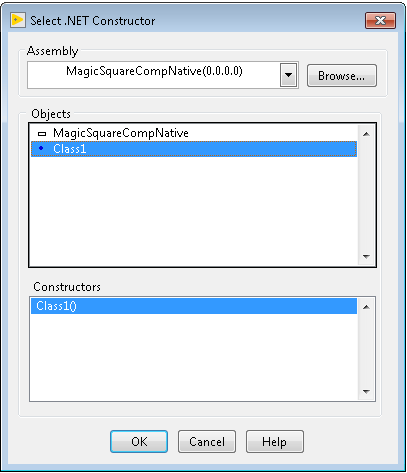1. From the Connectivity >> .NET pallet, add an Invoke Node(.NET) to the block diagram. Invoke Node invokes a method or action on a reference.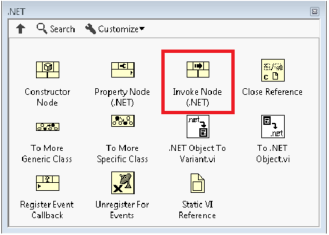1. Wire the output reference of the Constructor Node to the input reference of the Invoke Node. From the dropdown of the Invoke Node, select makesquare(Int32 numArgsOut, Object x) function.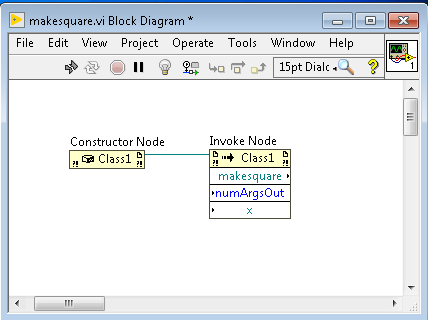1. “numArgsOut” is the number of output arguments to return. Since makesquare function only returns one output, set this input to a constant of 1.
1. Input “x” is a .NET object. Use the Connectivity >> .NET >> To .NET Object.vi to convert input data type to a .NET object.
2. Add a numeric array to your front panel. On the block diagram, wire the array control into the To .NET Object input.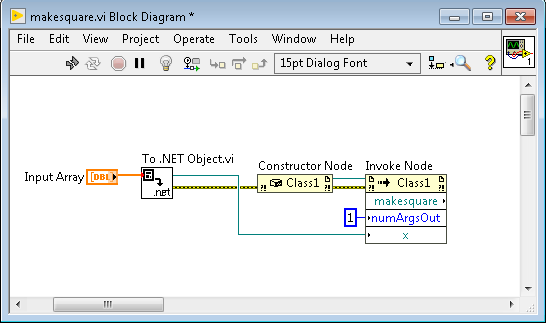1. Output “makesquare” is a .NET object that needs to convert to a variant. Use Connectivity >> .NET >>.NET Object To Variant.vi and Cluster, Class, and Variant >>Variant >> Variant to Data.VI. Configure the Variant to Data.VI to output a numeric result.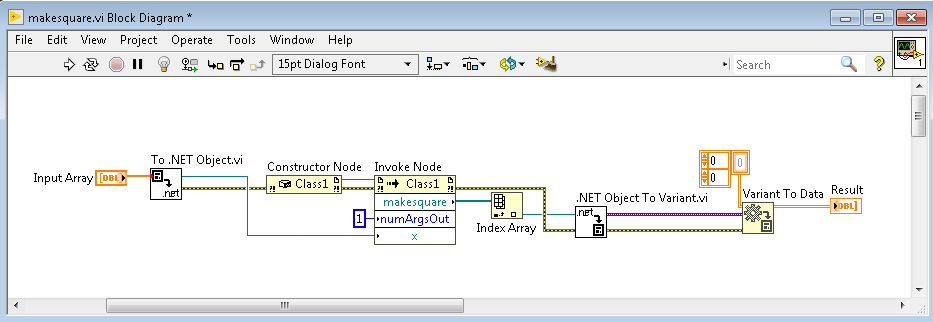1. Close .NET Reference with the Connectivity >> .NET >> Close Reference.VI.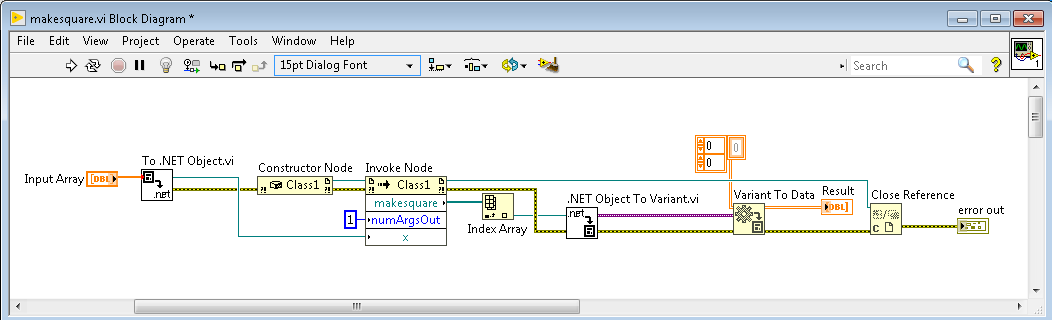The Front Panel should look like the following VI: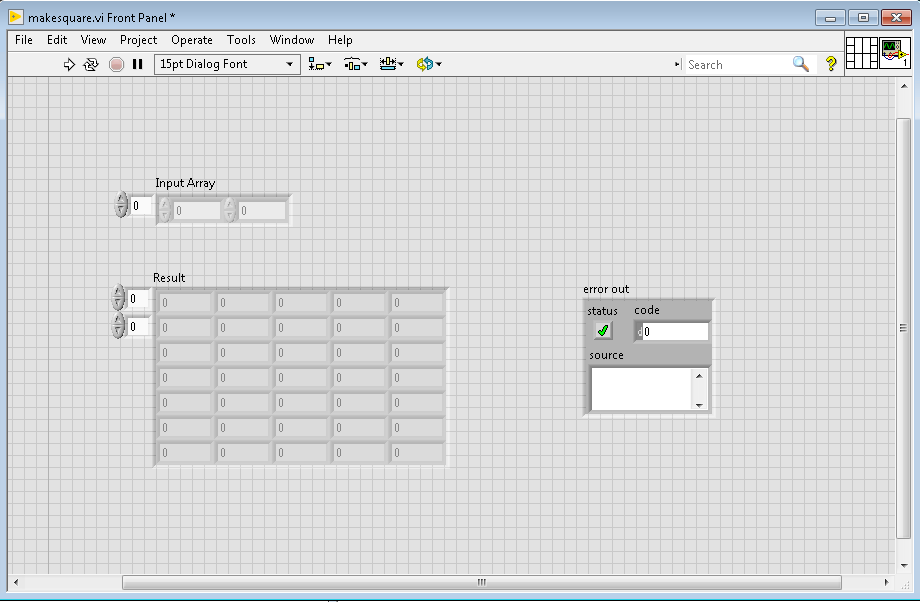Test this application by assign input array of value 5. Below is the result.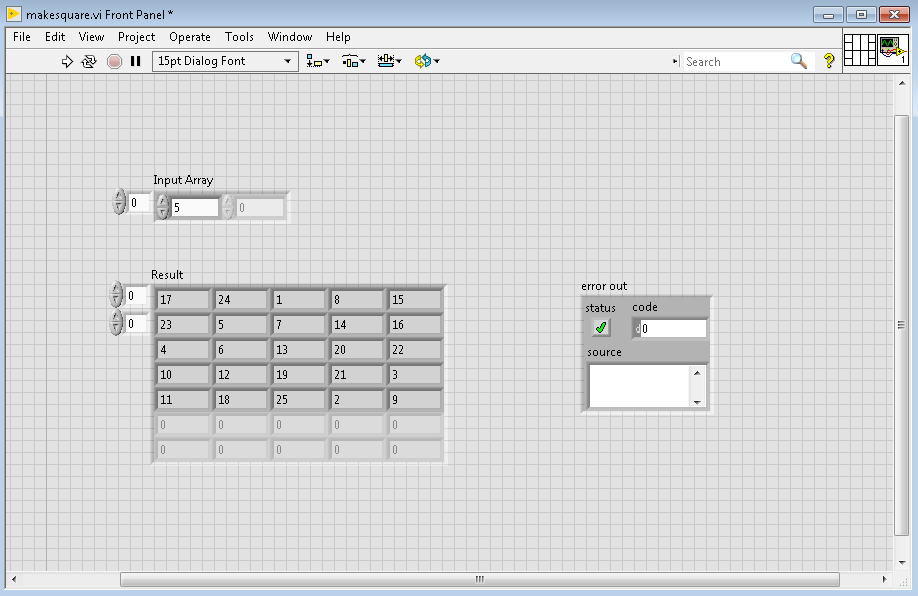Compare this result with the result from MATLAB’s makesquare.m.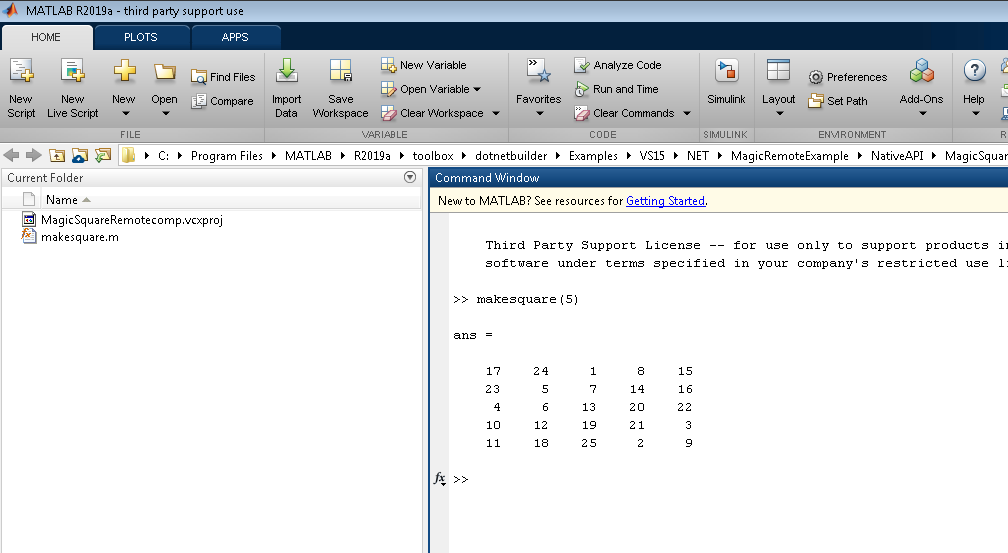Note on benchmark testing: In benchmark testing using the tic toc method, the MATLAB .NET library call was 1,783% faster than running the same code in LabVIEW MathScript Node and 16% faster compared to bundling the MATLAB code as an EXE and calling in with the LabVIEW exec.vi. The execution time for testing depends many factors such as the code being executed and computer's processing power. You can benchmark your own code by following the steps in Benchmarking Sections of Code in LabVIEW.

Other information on interfacing MATLAB and LabVIEW

MATLAB®, MATLAB Compiler™, and MATLAB Compiler SDK™ are trademarks or registered trademarks of The MathWorks, Inc.

### Ratings

Rate this document